Worksheet - Comparing Quantities

# Worksheet - Comparing Quantities - Mathematics (Maths) Class 7

Q.1. Find the ratio of (in lowest from) :
(a) 200g to 4 Kg
(b) 80 Paise to  ₹2
(c) 30 days to 36 hours

Ans. (a) 1 : 20
(b) 2 : 5
(c) 20 : 1

Q.2. If 3 : x :: 9 : 15 then find the value of x.

Ans. x = 5

Q.3. Express the following percents as the decimal fraction : (a) 0.07%
(b) 15%

Ans. (a) 0.0007
(b) 0.15

Q.4. Express each of the following percents as fraction in the simplest form :
(a)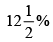(b) 75%

Ans.

(a)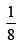(b)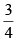Q.5. Express each of the following fractions as percent :

(a)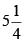(b)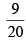Ans. (a) 525%
(b) 45%

Q.6. Find 20% of  ₹2500.

Ans. ₹500

Q.7. Find the whole quantity if 40% of it is 500 km

Ans. 1250 km

Q.8. In the market cost of 1 dozen bananas are ₹48. Find the cost of 10 bananas.

Ans. ₹40

Q.9. If the ratio of angles of a triangle is 2:3:4, then find the measure of these angles.

Ans. 40°, 60°, 80°

Q.10. If 75% of x is 18 then find the value of x.

Ans. x = 24

Q.11. Chalk contains Calcium, Carbon and Oxygen in the ratio 10:3:12. Find the percentage of Carbon in chalk.

Ans. 12%

Q.12. In a city, 35% are females, 45% are males and remaining are children. What % are children?

Ans. 20%

Q.13. Compute the Simple Interest on a sum of ₹ 900 for 5 years at the ratio of 6% per annum.

Ans. ₹270

Q.14. The cost price of 8 books is equal to the selling price of 6 books. Find the gain percent.

Ans.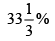Q.15. What principal will amount to ₹ 900 in 6 years at 10% Simple Interest?

Ans. ₹562.50

Q.16. There are 120 children live in Seemapuri 'J' Black. Accorching to servery conducted in 2014, out of these 24 children do not go to school" Under the project "School Chale Hum" these students admitted in school on the basis of above statement answer the following questions.
(a) In 2014, how many children did not go to school?
(b) Under the Project "School Chale Hum" How many children admitted in school.

Ans. (a) 20%
(b) 100%

The document Worksheet - Comparing Quantities | Mathematics (Maths) Class 7 is a part of the Class 7 Course Mathematics (Maths) Class 7.
All you need of Class 7 at this link: Class 7

## Mathematics (Maths) Class 7

121 videos|351 docs|45 tests

## FAQs on Worksheet - Comparing Quantities - Mathematics (Maths) Class 7

 1. What is the concept of comparing quantities in mathematics?Ans. Comparing quantities is a mathematical concept that involves analyzing and determining the relationship between two or more quantities. It helps in understanding the differences, similarities, and relative values of these quantities.
 2. How can we compare two quantities using ratios and proportions?Ans. Ratios and proportions are used to compare two quantities. A ratio is the comparison of the sizes of two or more quantities, while a proportion is an equation that states two ratios are equal. By setting up and solving proportions, we can compare the values of different quantities.
 3. What are the different methods of comparing quantities?Ans. There are several methods to compare quantities, such as using ratios, proportions, percentages, or graphical representations. Each method has its own advantages and is used depending on the nature of the quantities being compared and the context of the problem.
 4. How can we compare quantities in real-life situations?Ans. Comparing quantities is useful in real-life situations, such as comparing prices of products, determining discounts or markups, calculating interest rates, analyzing sales data, or evaluating cost-benefit ratios. By comparing quantities, we can make informed decisions and understand the relative value of different options.
 5. What are some common mistakes to avoid when comparing quantities?Ans. When comparing quantities, it is important to avoid common mistakes, such as comparing different units, comparing percentages without considering the base value, neglecting to convert units when necessary, or relying solely on one method of comparison without considering other relevant factors. It is crucial to carefully analyze the given information and consider all relevant factors to make accurate comparisons.

## Mathematics (Maths) Class 7

121 videos|351 docs|45 testsExplore Courses for Class 7 examSignup to see your scores go up within 7 days! Learn & Practice with 1000+ FREE Notes, Videos & Tests.
10M+ students study on EduRev
Track your progress, build streaks, highlight & save important lessons and more!
Related Searches

,

,

,

,

,

,

,

,

,

,

,

,

,

,

,

,

,

,

,

,

,

;